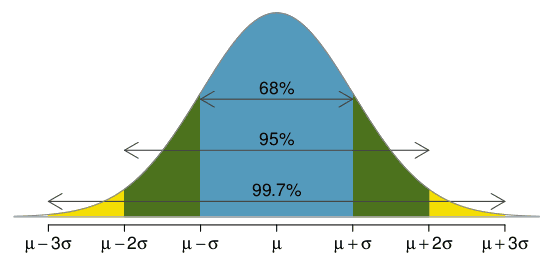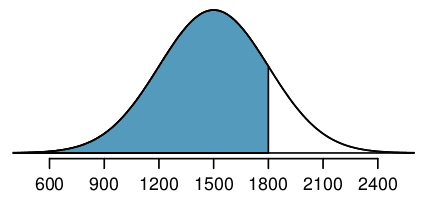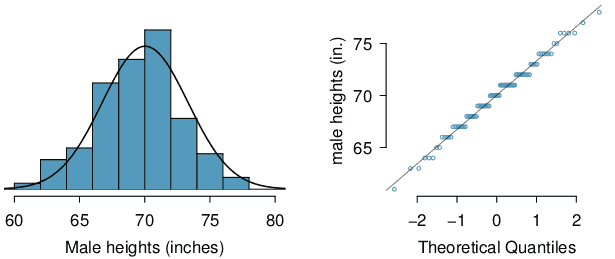# ML Wiki

## Normal Distribution

This is a continuous Symmetric, unimodal bell-shaped Distribution

• it has two parameters: mean $\mu$ and std $\sigma$, denoted as $N(\mu, \sigma)$
• Standard Normal Distribution is $N(\mu = 0, \sigma = 1)$

### Probability Density Function

x = seq(from=-3, to=3, length=15)
normalDensity = dnorm(x, mean=0, sd=1)
r = round(normalDensity, 2)
bp = barplot(r)
xspline(x=bp, y=r, lwd=2, shape=1, border="blue")
text(x=bp, y=r+0.03, labels=as.character(r), xpd=TRUE, cex=0.7)


Code## Z-score

### 68-95-99.7 rule

Also referred as the "rule of 3 sigmas"

• most of the data lay within 3 $\sigma$s from $\mu$
•### $Z$-score

$Z$-score of an observation is the number of standard deviations for the mean

• 1 sdt above - $z = +1$
• 1.5 std below - $z = -1.5$
• $z = \cfrac{x - \mu}{\sigma}$

we can use $z$-scores to identify unusual observations

• $x_1$ is more unusual than $x_2$ if $| z_1 | > | z_2 |$

### $Z$-standardization

• so $Z$-scores are used to standardize the observations
• in effect, it normalizes any normal distribution $N(\mu, \sigma$) to $N(0, 1)$
• see Normalization

### Percentile

Example:

• Scores of SAT takers are distributed normally
• parameters: $\mu = 1500, \sigma = 300$
• Ann earned 1800 on SAT,
• so Ann's $z = 1$

Ann's percentile - percent of people who earned lower SAT score

•• shaded - individuals who scored below Ann
• so knowing the $z$-score we can calculate the percentile
• Ann is the 84th percentile of SAT takers
• and vise-versa: we can also find $z$-score for given percentile

Example 2

• Shannon is a randomly selected SAT-taker.
• What's the probability that she'll score 1630 or more?
• Can find the $z$-score for that - it's $z = \cfrac{x - \mu}{\sigma} = 0.43$
• so we calculate the percentiles
• probability of getting below $z=0.43$ is 2/3
• so probability of getting above $z=0.43$ is 1 - 2/3 = 1/3

Always draw the bell shape first and then shade the area of interest

### $Z$-scores for Inferential Statistics

it may be useful for

## Normal Approximation

Many processes can be approximated well by normal distribution

• e.g. SAT, height of USA males, etc

But need to check if it's reasonable to use the normal approximation

2 visual methods for checking the assumption of normality

1. simple histogram + best fit of normal shape
•2. Q-Q Plot (or Normal Probability Plot)
•Code to produce the first figure:

load(url("http://www.openintro.org/stat/data/bdims.RData"))
fdims = subset(bdims, bdims$sex == 0) hist(fdims$hgt, probability=TRUE, ylim=c(0, 0.07))
x = 140:190
y = dnorm(x=x, mean=mean(fdims$hgt), sd=sd(fdims$hgt))
lines(x=x, y=y, col="blue")


Code to produce Q-Q Plots

qqnorm(fdims$hgt, col="orange", pch=19) qqline(fdims$hgt, lwd=2)